# Direct sum

(Redirected from Discrete direct product)

A construction extensively used in theories of mathematical structures which form categories similar to an Abelian category. In the non-Abelian case the direct sum is usually called the discrete direct product. Letbe a class of single-sorted algebraic systems which contain the one-element (zero) subsystems. The direct sum, or (discrete) direct product, of systems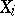,, inis the subsystem of the direct productconsisting of those functions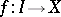for which all values, except for a finite number, belong to the corresponding zero subsystem. A direct sum is denoted by one of the following symbols:For a finite number of terms one also uses the notation: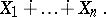The coincidence of the direct sum and the direct product in the case of a finite number of terms follows immediately from the definitions.

For each term of a direct sum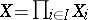there exists a canonical imbedding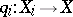that assigns to an elementthe function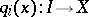, where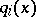takes the valueat the argumentand vanishes elsewhere. Hence it can be said that a direct sum contains its terms. In the case of an-group (in particular, for groups, Abelian groups, vector spaces, and rings) one can give an "intrinsic" characterization of a direct sum. An-groupis the direct sum of a family of-subgroups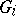,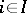, if: a)is generated by the,; b) eachis an ideal in; and c) the intersection ofwith the-subgroup generated by the remaining ideals is the trivial subgroup, for each. Cf. also Multi-operator group.

Every vector space is a direct sum of one-dimensional subspaces. Every free Abelian group is a direct sum of infinite cyclic groups. Every finite Abelian group is a direct sum of cyclic groups of prime-power order. Every semi-simple associative ring with a unit element and satisfying the minimum condition for ideals is the direct sum of a finite number of complete rings of linear transformations of appropriate finite-dimensional vector spaces.

In the theories of groups, lattices and categories, the isomorphism problem for direct decompositions has been extensively developed. Its origin is in the Remak–Schmidt theorem on central isomorphism of direct decompositions of groups having a principal series (cf. Krull–Remak–Schmidt theorem).

In category theory, the concept dual to that of a product, i.e. that of a coproduct, is sometimes called the direct sum.

In category theory the direct sum or coproduct is defined by a universal property: Given objects,, in a category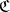. The direct sumis an object oftogether with morphisms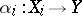such that for each objectofand family of morphisms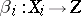there is a unique morphism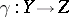such thatfor all. In many categories, e.g. those of Abelian groups and modules over a ring, the categorical direct sum is given by the construction outlined above.